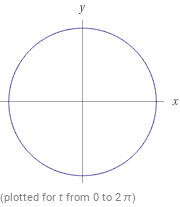Search IntMath
Close

450+ Math Lessons written by Math Professors and Teachers

5 Million+ Students Helped Each Year

1200+ Articles Written by Math Educators and Enthusiasts

Simplifying and Teaching Math for Over 23 Years

# Exploring Parts of a Circle in GeometryGeometry is a fascinating field of mathematics, full of interesting shapes, angles, lines, and curves. Among these shapes is the circle, or perhaps the most iconic of all the shapes in geometry. Circles are a fundamental part of geometry and understanding the different parts of a circle is essential for mastering the subject. In this article, we will explore the different parts of a circle and how they relate to one another.

The circle radius is one of the most important parts of a circle. It is the distance from the center of the circle to any point on the circle. The radius is always the same for any given circle, regardless of the size of the circle. This means that a small circle and a large circle have the same radius, just different lengths.

The radius is also used to calculate the circumference of a circle. The circumference is the total length of the outside of a circle, and it is calculated by multiplying the radius by 2 times pi (p). The equation for this is C = 2pr, where C is the circumference and r is the radius.

## Arc Length

Arc length is the measure of a curved line, or the length of a portion of the circumference of a circle. Arc length is calculated by multiplying the central angle of the arc (in radians) by the radius.

The central angle is the angle formed by two radii, or lines extending from the center of the circle to two points on the circle. The central angle is measured in degrees, and it is related to the arc length by the equation L = r?, where L is the arc length, r is the radius, and ? is the central angle in radians.

## Circle Sectors

A circle sector is a portion of a circle that is bounded by two radii and an arc. It is also known as a pie slice and is used to calculate the area of the sector. The area of a circle sector is calculated by multiplying the radius by the central angle in radians and then multiplying by one half (1/2). The equation for this is A = 1/2r?, where A is the area and r is the radius.

The area of a sector can also be used to calculate the area of a circle. The area of a circle is calculated by multiplying the radius by the radius and then multiplying by pi (p). The equation for this is A = pr2, where A is the area and r is the radius.

## Circumference and Area of a Circle

The circumference and area of a circle are related to each other. The circumference is the total length of the outside of a circle and it is calculated by multiplying the radius by 2 times pi (p). The area of a circle is calculated by multiplying the radius by the radius and then multiplying by pi (p). The equations for these are C = 2pr and A = pr2 respectively.

## Practice Problems

Let's practice what we've learned about the parts of a circle with some practice problems.

• If the radius of a circle is 3 cm, what is the circumference?
• If the radius of a circle is 8 cm, what is the area?
• If the central angle of a circle sector is 60�, what is the area of the sector?
• If the arc length of a circle is 12 cm, what is the central angle?
• If the circumference of a circle is 18p cm, what is the radius?

• The circumference of a circle with a radius of 3 cm is 18p cm.
• The area of a circle with a radius of 8 cm is 64p cm2.
• The area of a circle sector with a central angle of 60� is 16p cm2.
• The central angle of a circle with an arc length of 12 cm is 36�.
• The radius of a circle with a circumference of 18p cm is 3 cm.

Now that we have explored the different parts of a circle and practiced some problems, we can better understand the relationship between the radius, arc length, central angle, circumference, and sector area of a circle in geometry.

## Summary

In this article, we explored the different parts of a circle in geometry. We discussed the circle radius, arc length, central angle, circumference, and sector area. We also practiced solving problems related to these different parts of a circle. Understanding the parts of a circle is essential for mastering geometry, and we hope that this article has helped to make the topic easier to understand.

## FAQ

### What are the parts of circle in geometry?

The parts of a circle in geometry are the center point, radius, diameter, circumference, chord, arc, and sector.

### What are the 7 parts of a circle?

The 7 parts of a circle are the center point, radius, diameter, circumference, chord, arc, and sector.

### How you identify the parts of the circle?

The parts of a circle can be identified by looking at the shape of the circle and measuring different points, such as the radius, diameter, and circumference. Other parts, such as the chord, arc, and sector, can be identified by drawing lines and angles within the circle.